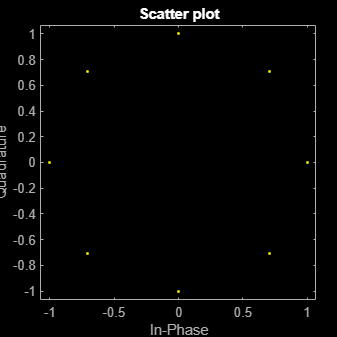# comm.PSKTCMModulator

Convolutionally encode binary data and map using M-ary PSK signal constellation

## Description

The `PSKTCMModulator` object implements trellis-coded modulation (TCM) by convolutionally encoding the binary input signal and then mapping the result to a PSK signal constellation.

To modulate a signal using trellis-coded modulation:

1. Define and set up your PSK TCM modulator object. See Construction.

2. Call `step` to modulate the signal according to the properties of `comm.PSKTCMModulator`. The behavior of `step` is specific to each object in the toolbox.

### Note

Starting in R2016b, instead of using the `step` method to perform the operation defined by the System object™, you can call the object with arguments, as if it were a function. For example, ```y = step(obj,x)``` and `y = obj(x)` perform equivalent operations.

## Construction

`H = comm.PSKTCMModulator` creates a trellis-coded M-ary phase shift keying (PSK TCM) modulator System object, `H`. This object convolutionally encodes a binary input signal and maps the result to an M-PSK constellation.

`H = comm.PSKTCMModulator(Name,Value)` creates a PSK TCM encoder object, `H`, with each specified property set to the specified value. You can specify additional name-value pair arguments in any order as (`Name1`,`Value1`,...,`NameN`,`ValueN`).

`H = comm.PSKTCMModulator(TRELLIS,Name,Value)` creates a PSK TCM encoder object, `H`. This object has the `TrellisStructure` property set to `TRELLIS` and the other specified properties set to the specified values.

## Properties

 `TrellisStructure` Trellis structure of convolutional code Specify trellis as a MATLAB® structure that contains the trellis description of the convolutional code. Use the `istrellis` function to check whether a trellis structure is valid. The default is the result of `poly2trellis`(```[1 3], [1 0 0; 0 5 2]```). `TerminationMethod` Termination method of encoded frame Specify the termination method as one of `Continuous` | `Truncated` | `Terminated`. The default is `Continuous`. When you set this property to `Continuous`, the object retains the encoder states at the end of each input vector for use with the next input vector. When you set this property to `Truncated`, the object treats each input vector independently. The encoder is reset to the all-zeros state at the start of each input vector. When you set this property to `Terminated`, the object treats each input vector independently. However, for each input vector, the object uses extra bits to set the encoder to the all-zeros state at the end of the vector. For a rate K/N code, the `step` method outputs the vector with a length given by $y=N×\left(L+S\right)}{K}$, where S = constraintLength–1 (or, in the case of multiple constraint lengths, S = sum(constraintLength(i)–1)). L indicates the length of the input to the `step` method. `ResetInputPort` Enable modulator reset input Set this property to `true` to enable an additional input to the `step` method. The default is `false`. When this additional reset input is a nonzero value, the internal states of the encoder reset to initial conditions. This property applies when you set the `TerminationMethod` property to `Continuous`. `ModulationOrder` Number of points in signal constellation Specify the number of points in the signal constellation used to map the convolutionally encoded data as a positive integer scalar value equal to `4`, `8`, or `16`. The default is `8`. The value of the `ModulationOrder` property must equal the number of possible output symbols from the convolutional encoder of the PSK TCM modulator. Thus, the value for the `ModulationOrder` property must equal 2N for a rate K/N convolutional code. `OutputDataType` Data type of output Specify the output data type as one of `double` | `single`. The default is `double`.

## Methods

 reset Reset states of the PSK TCM modulator object step Convolutionally encode binary data and map using M-ary PSK constellation
Common to All System Objects
`release`

Allow System object property value changes

## Examples

collapse all

Modulate random data using 8-PSK TCM modulation and display the constellation diagram.

Create binary data.

`data = randi([0 1],1000,1);`

Define a trellis structure with four input symbols and eight output symbols.

`t = poly2trellis([5 4],[23 35 0; 0 5 13]);`

Create an 8-PSK TCM modulator object using the trellis structure variable, `t`.

`hMod = comm.PSKTCMModulator(t,'ModulationOrder',8);`

Modulate and plot the data.

```modData = step(hMod,data); scatterplot(modData);```## Algorithms

This object implements the algorithm, inputs, and outputs described on the M-PSK TCM Decoder block reference page. The object properties correspond to the block parameters.